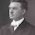## 23 January 2012

### 1/998001 generates a fascinating series

```1/998001 = 0.000001002003004005006007008009010011012013014015016017018019020
0210220230240250260270280290300310320330340350360370380390400410420430440450
4604704804905005105205305405505605705805906006106206306406506606706806907007
1072073074075076077078079080081082083084085086087088089090091092093094095096
0970980991001011021031041051061071081091101111121131141151161171181191201211
2212312412512612712812913013113213313413513613713813914014114214314414514614
7148149150151152153154155156157158159160161162163164165166167168169170171172
1731741751761771781791801811821831841851861871881891901911921931941951961971
9819920020120220320420520620720820921021121221321421521621721821922022122222
3224225226227228229230231232233234235236237238239240241242243244245246247248
2492502512522532542552562572582592602612622632642652662672682692702712722732
7427527627727827928028128228328428528628728828929029129229329429529629729829
9300301302303304305306307308309310311312313314315316317318319320321322323324
3253263273283293303313323333343353363373383393403413423433443453463473483493
5035135235335435535635735835936036136236336436536636736836937037137237337437
5376377378379380381382383384385386387388389390391392393394395396397398399400
4014024034044054064074084094104114124134144154164174184194204214224234244254
2642742842943043143243343443543643743843944044144244344444544644744844945045
1452453454455456457458459460461462463464465466467468469470471472473474475476
4774784794804814824834844854864874884894904914924934944954964974984995005015
0250350450550650750850951051151251351451551651751851952052152252352452552652
7528529530531532533534535536537538539540541542543544545546547548549550551552
5535545555565575585595605615625635645655665675685695705715725735745755765775
7857958058158258358458558658758858959059159259359459559659759859960060160260
3604605606607608609610611612613614615616617618619620621622623624625626627628
6296306316326336346356366376386396406416426436446456466476486496506516526536
5465565665765865966066166266366466566666766866967067167267367467567667767867
9680681682683684685686687688689690691692693694695696697698699700701702703704
7057067077087097107117127137147157167177187197207217227237247257267277287297
3073173273373473573673773873974074174274374474574674774874975075175275375475
5756757758759760761762763764765766767768769770771772773774775776777778779780
7817827837847857867877887897907917927937947957967977987998008018028038048058
0680780880981081181281381481581681781881982082182282382482582682782882983083
1832833834835836837838839840841842843844845846847848849850851852853854855856
8578588598608618628638648658668678688698708718728738748758768778788798808818
8288388488588688788888989089189289389489589689789889990090190290390490590690
7908909910911912913914915916917918919920921922923924925926927928929930931932
9339349359369379389399409419429439449459469479489499509519529539549559569579
5895996096196296396496596696796896997097197297397497597697797897998098198298
3984985986987988989990991992993994995996997999 ...```
Start at the beginning, or pick a place in the middle and start reciting the numbers.  Someone out there can probably explain why.  At its extremes, mathematics devolves into a kind of magic...

Found in the Futility Closet, which has an abundance of math-related items.

1.1/998001 = (1/999)^2

1/999 = 0.001001001....

Sort of comes from there.

Now what is really strange is

23.140692632779269005729086367949... to the power of i (imaginary, i = sqrt(-1)) is -1.

1.Well, that's simple, 'cause e to the power of pi is equal to 23.140... and e to the power of iPi (is Apple behind all this?... holy mother of god... IT'S A CONSPIRACY!) in the Gaussian plain (Gaußsche Zahlenebene, sorry, I'm German) is just cos(pi), which is -1. It's not that strange. Only complex. :D

2.yep, that's it. You have to write a square matrix of 001's (999 rows and columns). The nth digit block of 1/998001 is given by the sum over the nth diagonal, i.e. n x 001. Try it with the easiest one, 1/9 = 0.11111, 1/81 = (1/9)^2 = 0.012345678901234...

3.sorry, the second last one is really always missing, e.g. for 1/81: 0.01234567901234...

2.I forwarded this item to a friend who replied, Is the result correct? So I found an online calculator which is up to the task, and the result agrees. http://www.math.jerrywickey.net/?prol=y&first=n&r=0.0e0&a=1&b=998001&cal=d&bp=3200+dec+places&do=Compute

1.Including this apparent glitch at the end?

992993994995996997999

I haven't looked for other irregularities.

2.It's not a roundoff thing: 998 really is missing, according to the calculation at the link in my comment above. After 999, the whole thing starts over with 000 and continues repeating forever.

3.I just realized this would make a nice bar bet. Write down the fraction, and then bet someone that you can calculate it to a hundred decimal places before they can do it to ten.

4.Yeah, you just have to remember what we were all taught in high school, (maybe earlier. (Really, I swear.)) that any repeating non terminating decimal is a rational number. If you start with the repeating number you want, you can recover its rational value. With a big enough calculator, in this case. Assign digit values to 'Minnesotastan' (or whatever), and you can find 'your' fraction.

5.Having some experience with numerical roundoff, I think the 'glitch' in the end is indeed that. That last 9 stands for 8999, which rounds of to '9'.

1.Well, that makes sense. Thanks.

2.I don't think that this is due to numerical errors, I rather think it's a systematic pattern.

As far as I can see, the second last number is always missing.
I have got no mathematical proof for it yet, but it is quite obvious:

For 1/998001 the 998 is missing.
For 1/9801 the 98 is missing.
For 1/81 the 8 is missing.

For 1/7601 in octal, the 76 is missing,
For 1/FE01 in hex, its FE.

I just wrote a small program which calculated the series using long devision,
if anyone wants to check it out: http://pastebin.com/i5Shcfda

6.Stan, thanks for this post. Heumpje, thanks for the clarification about the roundoff. I was wondering why it skipped a digit!

7.Mark above is exactly right. If you use 99^2=9801 instead, you get 0.0001020304..., and if you use 9^2=81, you get 0.01234567... If you use 9999^2=99980001, you get 0.00000001000200030004...

The reason is that the sum of nx^(n+1) from n=0 to infinity equals (1/x - 1)^(-2). (You can show this by checking they have the same derivative.) If you plug in x=1/1000 (say), the infinite sum becomes the decimal expansion you had, and it's value is 999^(-2).

A welcome chance for me to give back to TYWKIWDBI!

1.I am recurrently gobsmacked by the breadth and depth of knowledge of readers here. Thanks, James.

8.Oops! I should have said you can check the identity by showing the two sides become equal after you divide by x^2 and integrate.

9.998 isn't missing. The next number 999 'overflowed' into 998. Likewise the next number 1000, the 1 was added to 999.

10.Some people won't read. Some others can't think.

11.you have realized that this fraction and its decimal result briefly describe the initial terms of the Fibonacci sequence??????
What do you think??????????

12.there is nothing strange, nor glitch, it's mathematical. People! Stop! Stop giving emotional content over something which might look as a coincidence but which is not. There is no special god-alike hidden SMS in fraction. No number-encrypted magic serial number and no free-pass to matrix heaven.

Please, look at it rationally, with your logical brain. If I would tell you:

1-1 = 0

Would you ask me where is the 1? Same thing here.